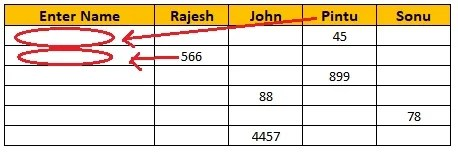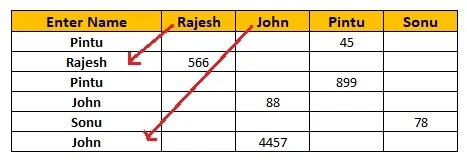# How to Select Automatic a Name from Column with Formula in Excel

Rate this post !

The table in Name and Sales amount to enter the salesman name automatically in a cell with the formula in excel

Raw DataOutput Data DataFormula: =IF(ISNUMBER(B3),\$B\$2,IF(ISNUMBER(C3),\$C\$2,IF(ISNUMBER(D3),\$D\$2,IF(ISNUMBER(E3),\$E\$2,””))))

Video Tutorial